## Thursday, February 18, 2016

### Removing Slow Variations from Signals with CDF(4, 4): Programmatic Note 13 on Ripples in Mathematics

Problem

This is my programmatic note 13 on Ch. 04, p. 31-34, in "Ripples in Mathematics" by A. Jensen & A. la Cour-Harbo. These notes document my programmatic journey, sometimes easy, sometimes painful but always joyful, through this great text. My notes are for those who want to use Java as a programming investigation tool of various wavelet algorithms and Octave for plotting the results. You can find my previous notes by searching my blog on "ripples in mathematics." If you are on the same journey, let me know of any bugs in my code or improvements you have found that let us all, fellow travelers on the wavelet road, get the results in a better way.

In this note, I document my programmatic reconstruction of the example that starts on p. 31 with the logarithm function in Eq. 4.1 on p. 31. The function is defined as f(t) = log(2 + sin(3*pi*sqrt(t)). The function is sampled 1024 times at points 1/1024, 2/1024, 3/1024, ..., 1024/1024. The values at 1/1024, 33/1024, 65/1024, etc. are set to 2. These are the fast variations.  The multiresolution analysis is used to remove slow variations from the signal. To put it differently, the multiresolution analysis allows us to design filters for fast variations.

Graphing Logarithmic Function in Equation 4.1

I abstracted the function in Eq. 4.1 into a Java class Ripples_F_p33.java.

public class Ripples_F_p33 extends Function {
public Ripples_F_p33() {}
@Override
public double v(double t) { return Math.log(2.0 + Math.sin(3 * Math.PI * Math.sqrt(t))); }

}

The values computed by this function were placed in an Octave script fig_4_13_p33.m. Fig. 1 shows the Octave graph of this function given on p. 33 in "Ripples."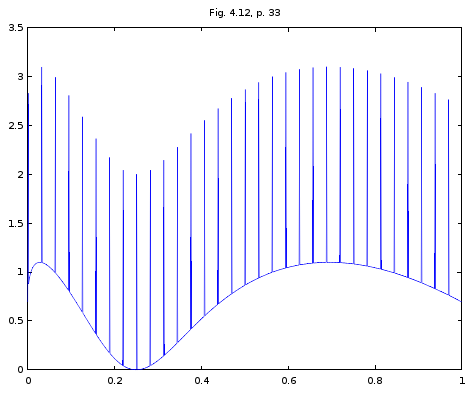Figure 1. Graph of f(t) = log(2 + sin(3*pi*sqrt(t)) with added variations at i/1024, for i = 1, 33, 65, ...

Multiresolution Analysis of Signal in Fig. 1

The removal of slow variations from the original signal starts with the multiresolution analysis detailed in this section. To begin with, I applied six scales of CDF(4, 4) to reconstruct the multi-resolution analysis in Fig. 4.13 on p. 34 in "Ripples." Below is a auxiliary static method I added to RipplesInMathCh04.java.

static void multires_fig_4_13_p34(String message, int range_start, int range_end) {
for(int i = 0; i < 1024; i++)  {
sRangeFig_4_12_p33[i] = sRipples_F_p33.v(sDomainFig_4_12_p33[i]);
}

for(int i = 1; i < 1024; i += 32) {
sRangeFig_4_12_p33[i] += 2;
}

CDF44.orderedDWTForNumIters(sRangeFig_4_12_p33, 6, false);

double[] signal = new double[sRangeFig_4_12_p33.length];
for(int i = 0; i < 1024; i++) {
if ( i >= range_start && i <= range_end ) {
signal[i] = sRangeFig_4_12_p33[i];
}
else {
signal[i] = 0;
}
}

System.out.println("=========================");
System.out.println(message);
display_signal(signal);
System.out.println("Inversed Signal");
System.out.println("=========================");
CDF44.orderedInverseDWTForNumIters(signal, 6, false);
display_signal(signal);
System.out.println("=========================");
}

Then I added several static methods that call multires_fig_4_13_p34(). Each function below is followed by the graph generated by the corresponding Octave scripts.

static void fig_4_13_D10_p34() {
multires_fig_4_13_p34("Fig. 4.13, 06-06-07-08-09-D10, p. 33", D10_START_1024, D10_END_1024);
}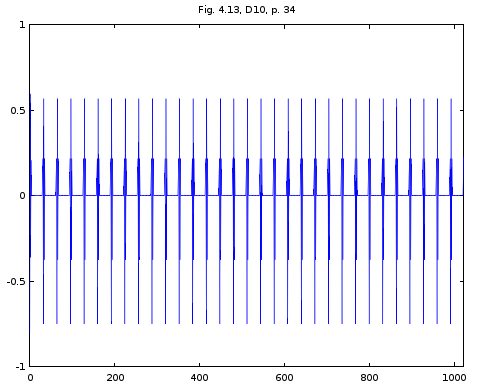Figure 2. Signal reconstructed from CDF(4,4) D10 wavelet coefficients generated by fig_4_13_p34_d10.m

static void fig_4_13_D9_p34() {
multires_fig_4_13_p34("Fig. 4.13, 06-06-07-08-D9-010, p. 33", D9_START_1024, D9_END_1024);
}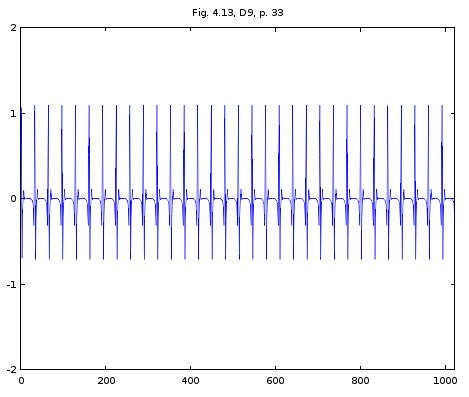Figure 3. Signal reconstructed from CDF(4,4) D9 wavelet coefficients generated by fig_4_13_p34_d9.m

static void fig_4_13_D8_p34() {
multires_fig_4_13_p34("Fig. 4.13, 06-06-07-D8-09-010, p. 33", D8_START_1024, D8_END_1024);
}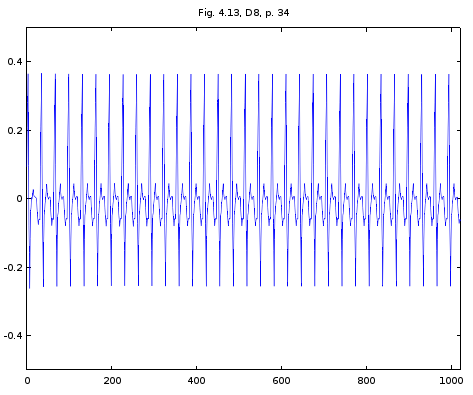Figure 4. Signal reconstructed from CDF(4,4) D8 wavelet coefficients generated by fig_4_13_p34_d8.m

static void fig_4_13_D7_p34() {
multires_fig_4_13_p34("Fig. 4.13, 06-06-D7-08-09-010, p. 33", D7_START_1024, D7_END_1024);
}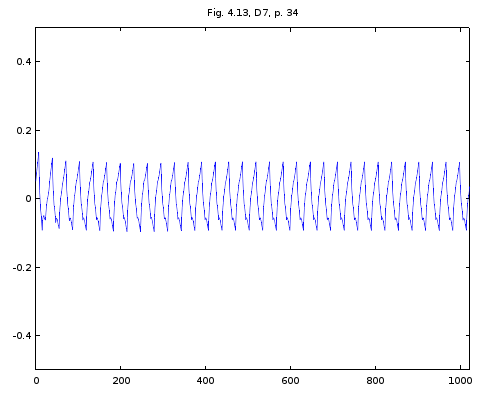Figure 5. Signal reconstructed from CDF(4,4) D7 wavelet coefficients generated by fig_4_13_p34_d7.m

static void fig_4_13_D6_p34() {
multires_fig_4_13_p34("Fig. 4.13, 06-D6-07-08-09-010, p. 33", D6_START_1024, D6_END_1024);
}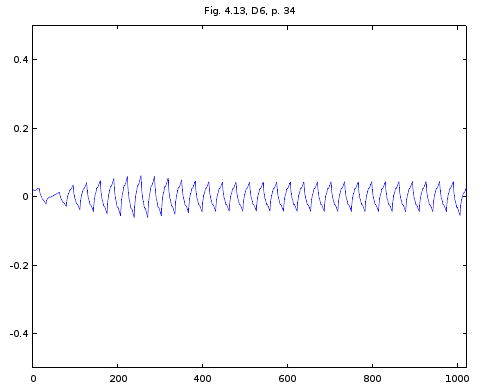Figure 6. Signal reconstructed from CDF(4,4) D6 wavelet coefficients generated by fig_4_13_p34_d6.m

static void fig_4_13_S6_p34() {
multires_fig_4_13_p34("Fig. 4.13, S6-06-07-08-09-010, p. 33", S6_START_1024, S6_END_1024);
}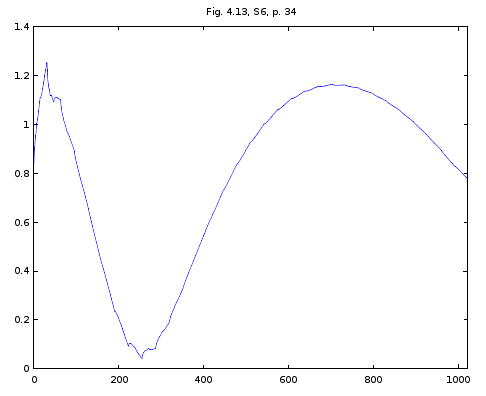Figure 7. Signal reconstructed from CDF(4,4) S6 wavelet coefficients generated by fig_4_14_s6_p35.m

Removing Slow Variations from 1D Signal in Fig. 1

I implemented a separate class, ApplyDWT.java, with a method keepFastVariationsInSignal(). This method implements a fast variation signal filter. There are four logical steps commented in the code below: 1) apply the DWT to the signal for the required number of scales; 2) remove the slow variations from the signal into a separate 1D array; 3) reconstruct the signal from the array with the slow variations obtained in step 2; 4) subtract the reconstructed signal obtained in step 3 from the original signal. This reconstructed signal will contain only the fast variations because the slow variations will be subtracted away.

public static void keepFastVariationsInSignal(double[] signal, ApplyDWT.DWT dwt, int num_iters, int range_start, int range_end) {
// signal_transform holds the DWT transform of signal_tranform of ApplyDWT.DWT
double[] signal_transform           = new double[signal.length];
// - signal_with_filtered_range holds the slow variations filtered from the signal
// transform in the required range [range_start, range_end];
// - the reconstructed signal is reconstructed from filtered_range_from_signal_transform.

double[] filtered_range_from_signal_transform = new double[signal.length];

// 0. copy signal into signal_transform
System.arraycopy(signal, 0, signal_transform, 0, signal_transform.length);
// 1. apply forward DWT to signal
forwardDWTForNumIters(signal_transform, dwt, num_iters, range_start, range_end);
// 2. filter the required range from signal transform into filtered_range_from_signal_transform;
//    filtered_range_from_signal_transform contains slow variations.

filterSignalRange(signal_transform, filtered_range_from_signal_transform, range_start, range_end);
// 3. reconstruct signal from slow variations for the same number of iterations
inverseDWTForNumIters(filtered_range_from_signal_transform, dwt, num_iters);
// 4. subtract the reconstructed signal from the original signal to keep the fast variations;
//    fast variations = original signal - signal reconstructed from slow variations.

subtractSignals(signal, filtered_range_from_signal_transform);
filtered_range_from_signal_transform = null;
signal_transform           = null;
}

The static method below in RipplesInMathCh04.java generates the sample values for the graph in Fig. 4.15 on p. 35 in "Ripples."
static void fig_4_15_cdf44_p35() {
for(int i = 0; i < 1024; i++)  {
sRangeFig_4_12_p33[i] = sRipples_F_p33.v(sDomainFig_4_12_p33[i]);
}

for(int i = 1; i < 1024; i += 32) {
sRangeFig_4_12_p33[i] += 2;
}

ApplyDWT.keepFastVariationsInSignal(sRangeFig_4_12_p33, ApplyDWT.DWT.CDF44, 5, S6_START_1024, S6_END_1024);

display_signal(sRangeFig_4_12_p33);
System.out.println("=========================");

}

Below is the graph generated by the Octave script fig_4_15_p35.m from the values returned by fig_4_15_cdf44_p35()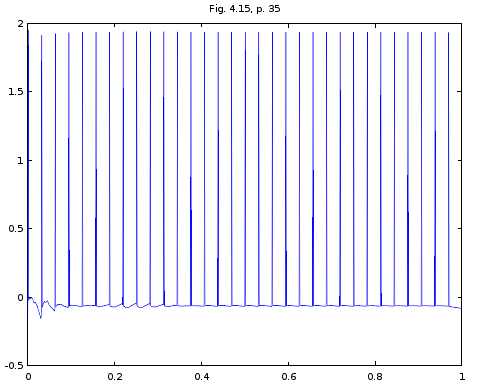Figure 8. Fast variations from filtered from signal in Fig. 1